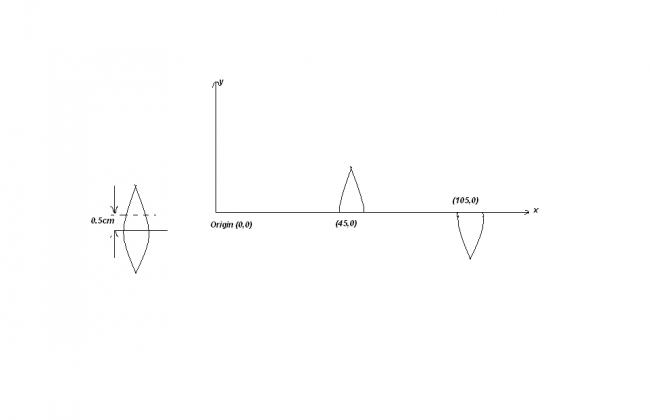# >> LENSES <<

A thin convex lens whose focal length in air is known to be 30cm is cut into two parts by a transverse plane parallel to principal axis and 0.5cm above the principal axis. They are placed on the x-axis a shown in the figure.A point object is placed on the origin of the coordinate system.Find the co-ordinates of the final image formed due to the rays which get refracted by both the parts of the lens (assuming paraxial ray approximations to be valid)•akshay sharma ·

pls help me guys.....

•akshay sharma ·

I was going in the same way as urs ......

but buddy answer given is that final image is formed at (120cm, -1cm)

•Avik ·

Oh yeah, i made big mistakes :P

Akshay, which part of the lens is placed where....Is it given ?

•akshay sharma ·

nopes !!

i hav mentioned all the data above which is given in the question .

nothing else is given... bro.

•akshay sharma ·

till now no one?????

•rahulxj100 ·

1/v - 1/(-45)=1/30
therefore v will be 90.....
now this will act as an object for the second lens

1/v - 1/15=1/(-30)
u will get v as 30

therefore the image will be formed at (90+30)=120.....

(i think this is the answer)

•Tirthankar Bhattacharya ·

The -1 can be calculated by finding out the magnifications on consecutive refractions-0.5-0.5

•